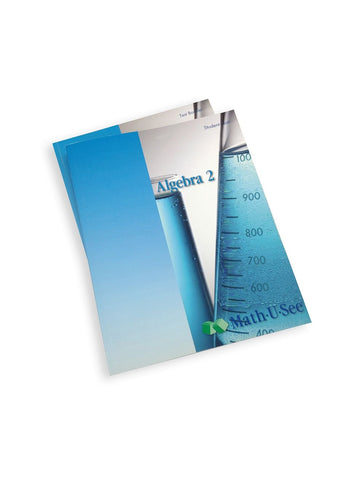# Math-U-See Algebra 2 Student Pack

• \$ 48.00

#### Algebra 2 Student Pack contains the Student Workbook with lesson-by-lesson worksheets, review pages, and honors pages. It also includes the Algebra 2 Tests. .

Factoring Polynomials, Quadratic Formula, Graphing Conic Sections, and Other Algebra 2 Topics

Algebra 2 is a more rigorous algebra course that builds on the previous two courses. Besides review and expansion of topics taught in Algebra 1, the course covers advanced factoring, imaginary and complex numbers, conjugate numbers, the binomial theorem, the quadratic formula, motion problems, and other kinds of application problems. Graphing includes extensive practice with conic sections and solving systems of equations visually and algebraically. Vectors are also introduced.